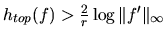## Large topological entropy implies existence of a maximal entropy measure for interval maps

Discrete Contin. Dyn. Syst. Ser. A, 14 (4), 673-688, 2006.

### Abstract

We give a new type of sufficient condition for the existence of measures with maximal entropy for an interval map f, using some non-uniform hyperbolicity to compensate for a lack of smoothness of f. More precisely, if the topological entropy of a C1 interval map is greater than the sum of the local entropy and the entropy of the critical points, then there exists at least one measure with maximal entropy. As a corollary, we obtain that any C r interval map f such thatpossesses measures with maximal entropy.

Paper: [arXiv:1901.01073] [pdf (published paper)]